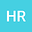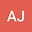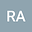Lie analysis, conserved quantities and solitonic structures of Calogero-Degasperis-Fokas equation
•••• +2
University of Guilan

Corresponding Author:[email protected]

Author ProfileReza Abazari
Author ProfileKenan Yildirim
Mus Alparslan Universitesi
Author ProfileSumaira Sharif
University of Central Punjab
Author Profile## Abstract

The paper investigates a class of exactly solvable third order nonlinear evolution equation . A list of unknown function F(u) is reported for which considered equation contains the nontrivial Lie point symmetries. Moreover, nonlinear self-adjointness is discussed and it is examined that it is not strictly self-adjoint equation for physical parameter A 6= 0 but quasi self-adjoint or more generally nonlinear self-adjoint. Additionally, it is observed that Calogero-Degasperis-Fokas (CDF) equation admits a minimal set of Lie algebra under invariance criteria of Lie groups. These classes are utilized one by one to construct the similarity variables to reduce the dimension of the discussed equation. Additionally, Lie symmetries are used to exhibit the associated conservation laws. Henceforth, Lie symmetry reductions of CDF equation are reported with the help of an optimal system. Meantime, this Lie symmetry method reduces the considered equation into ordinary differential equations. Moreover, well known (G’/G)-expansion method is used to get the exact solutions. The obtained new periodic and solitary wave solutions can be widely used to provide many attractive complex physical phenomena in the different fields of sciences.